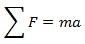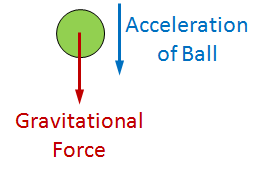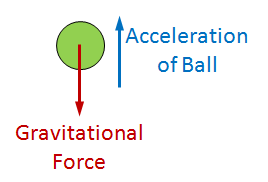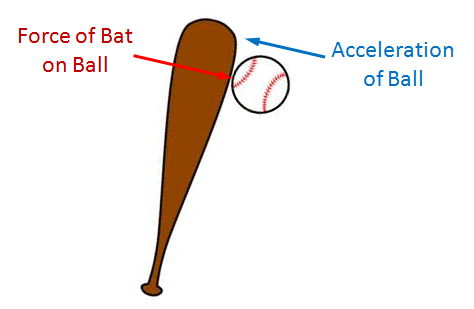# Negative Mass/Inertia

You have entered a negative mass or inertia. This simulation cannot handle negative masses of inertias because it assumes Newton’s laws of motion.

To see the problem, we will look at Newton’s second law which states that force is equal to mass times acceleration, i.e.,Under normal circumstances (if the mass is positive), this relation tells us that if a body is acted upon by a force, than the body will accelerate in the direction in which the force is applied. For example, if a ball is released above the ground, then gravity will pull down on it causing it to accelerate toward the ground.However, given the same situation excepting that the mass of the ball is negative, the ball will accelerate upwards.In this case, the result seems somewhat reasonable. However if we look at a different example, the problem becomes more apparent. In this example, we will look a bat hitting a ball. If the mass of the ball is negative in this case, the force of the bat acting on the ball will cause the ball to accelerate towards the bat. The problem is that the ball is already touching the bat, which means that to accelerate towards the bat; the ball would have to go through the bat, which cannot happen without completely destroying the ball and/or the bat.Because of this problem, this simulation cannot handle negative masses meaningfully.

Inertia is the rotational version of mass, so the same problems applies to negative inertias also.

 Units English (feet)English (inches)Metric Length of Short Arm ft Length of Long Arm ft Length of Sling ft Length of Weight ft Height of Pivot ft Uniform arm Mass of Arm lb Inertia of Arm lb·ft² Pivot to Arm CG ft Mass of Weight lb Inertia of Weight lb·ft² Projectile BaseballGolf BallPumpkinCowCustom Mass of Projectile lb Projectile Diameter ft Wind Speed ft/s Release Angle ° Play speed: 1x Link to save this trebuchet

## VirtualTrebuchet 2.0

VirtualTrebuchet is a web based trebuchet simulator that will allow you to quickly evaluate different trebuchet configurations.

1. To begin, enter the parameters of your trebuchet in the input boxes.
2. Next, press the "Simulate" button located under the inputs.
3. Finally, watch your trebuchet go.